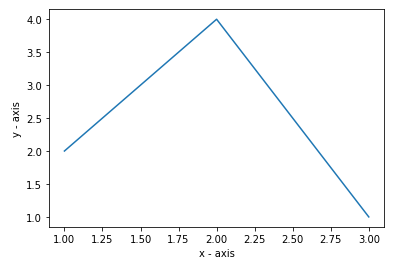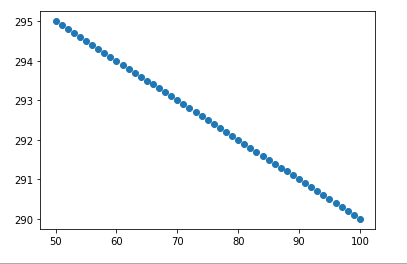Matplotlib.pyplot.autoscale() in Python

• Last Updated : 23 Jun, 2020

Matplotlib is a widely used library in Python for plotting various graphs, as it provides very efficient ways and easy to understand methods for complex plots also. matplotlib.pyplot is a plotting library used for 2D graphics in Python programming language. Pyplot is a collection of command style functions that make matplotlib work like MATLAB.

autoscale() function

matplotlib.pyplot.autoscale() is a method for simple axis view autoscaling. It turns autoscaling on or off, and then, if autoscaling for either axis is on, it performs the autoscaling on the specified axis or axes.

Syntax: matplotlib.pyplot.autoscale(enable=True, axis=’both’, tight=None)

Parameters:
enable is a Boolean valued parameter, if it is set to True the autoscaling is on else auto-scaling is off. It is an optional parameter. When not specified, it takes the default value as ‘true’.
axis is another optional parameter which states that axis to operate on. Usually it can be ‘both’, ‘x’, or ‘y’. When not specified, it takes default value as ‘both’.
tight takes the boolean argument, If True, first set the margins to zero. Then, this argument is forwarded to autoscale_view (regardless of its value). This is also an optional parameter of the autoscale method.

Example 1:

 # importing the required module import matplotlib.pyplot as plt   # x axis values x = [1,2,3]   # corresponding y axis values y = [2,4,1]   # plotting the points plt.plot(x, y)   # naming the x axis plt.xlabel('x - axis')   # naming the y axis plt.ylabel('y - axis')   plt.autoscale() plt.show()

Output:Example 2:

 import numpy as npimport matplotlib.pyplot as plt    x, y = np.arange(0, 101, 1), 300 - 0.1 * np.arange(0, 101, 1)mask = (x >= 50) & (x <= 100)  fig, ax = plt.subplots()ax.scatter(x[mask], y[mask])  plt.autoscale()plt.show()

Output:My Personal Notes arrow_drop_up